# Normalizing the Output of an FFT Routine

The DFT expression [4.3] doesn't have a clue about the original sampling rate used to form the discrete-time vector; therefore, it can't really compute the correct value of the Fourier transform without auxiliary scale factors. If you want your Fourier transformations to produce results with the correct amplitude, you must apply the scale factors every time you use either the forward or inverse transformation.

The mathematical spreadsheet package I use, called MathCad, provides a forward-transform FFT and its inverse IFFT defined as in [4.3] and [4.4]. When using these transformations in an environment with a sample interval of D T and a discrete-vector length of N , the appropriate scale factors work like this:

Equation 4.6Equation 4.7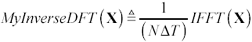Other tools may define their FFT routines differently, requiring different scaling constants (see Section 4.10, "Checking the Output of Your FFT Routine").

MathCad routines FFT( ) and its inverse IFFT( ) are specialized for working with real-valued data sequences (as opposed to sequences with complex values). A real-valued data sequence x n has the property that the FFT of that sequence, X k , is complex-conjugate-symmetric around the origin, which means in practical terms that if you know the output point X i , then you can trivially compute the output point X N-i . The MathCad functions FFT( ) and IFFT( ) therefore don't bother to generate, store, or use values of X k for k greater than N /2. Since the top half of each frequency-domain vector associated with FFT( ) and IFFT( ) is unnecessary, the length of X is truncated to ( N /2) + 1. The length of the time-domain vector x remains N .

4.5.1 Deriving the DFT Normalization Factors

When used as an approximation to the Fourier transform, the DFT requires a scaling factor. This scaling factor derives from [4.1], substituting for the integral an approximate summation carried out using the available samples taken at points in time t n = n · D T .

Equation 4.8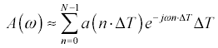Presuming that you wish only to compute values of the output on a dense grid of frequencies w k = 2 p k/(N · D T) , where k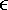0,1..( N “1),

Equation 4.9The sampled values of a ( t ) and A ( w ) may be represented in vector notation:

Equation 4.10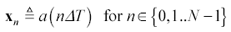Equation 4.11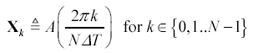which simplifies the appearance of [4.9]:

Equation 4.12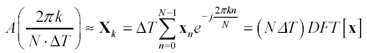Going in the other direction, suppose you have an expression for the Fourier transform A ( w ) of a waveform a ( t ). To approximate a ( t ) using the inverse DFT, you first sample the frequency-domain function A ( w ) according to [4.11], apply the inverse DFT to X , and then multiply by the scaling factor 1/(N · D T ).

Equation 4.13The scaling factors apply to a DFT of any length, although when using the Cooley-Tukey FFT algorithm the length N will always be a power of two.

POINT TO REMEMBER

• Most FFT routines require external scale factors that depend on the sample interval D T and sample vector length N .High-Speed Signal Propagation[c] Advanced Black Magic
ISBN: 013084408X
EAN: N/A
Year: 2005
Pages: 163